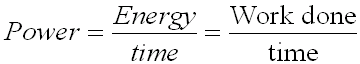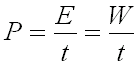#### Power

Power is the rate at which energy is used or the rate at which work is done.

Mathematically, the above statement can be expressed as:In symbols, this expression is written as:The unit of power is the WATT, (W)1 W = 1 J/s

Example:

A crane lifts a 250 Kg crate of roof shingles from the ground on top of a 15 m high roof  in 20 s.  How much power did the crane use up?

Given:

m = 350 Kg

g = 9.8 m/s2

h = 15 m

t =  20 s

Find:

a) Force required

b) Work Done

c) Power

Solution:

a)The force Required to Lift Shingles up is the force of gravity, F
Fg = m x g (the force of gravity)
= 350 Kg x 9.8 N/Kg
= 3430 N

b) Work Done = Work Done in lifting
= Fg x h
= 3430 N x 15 m
= 51 450 Nm
= 51 450 J

c) Power = Work / time
= W / t
= 51 450 J /  20 s
= 2573 W